# 第四次作业

【课程观看报告】

1、观看了三节内容，共计八个视频2、通过观看视频你学习到哪些知识点或者编程题目？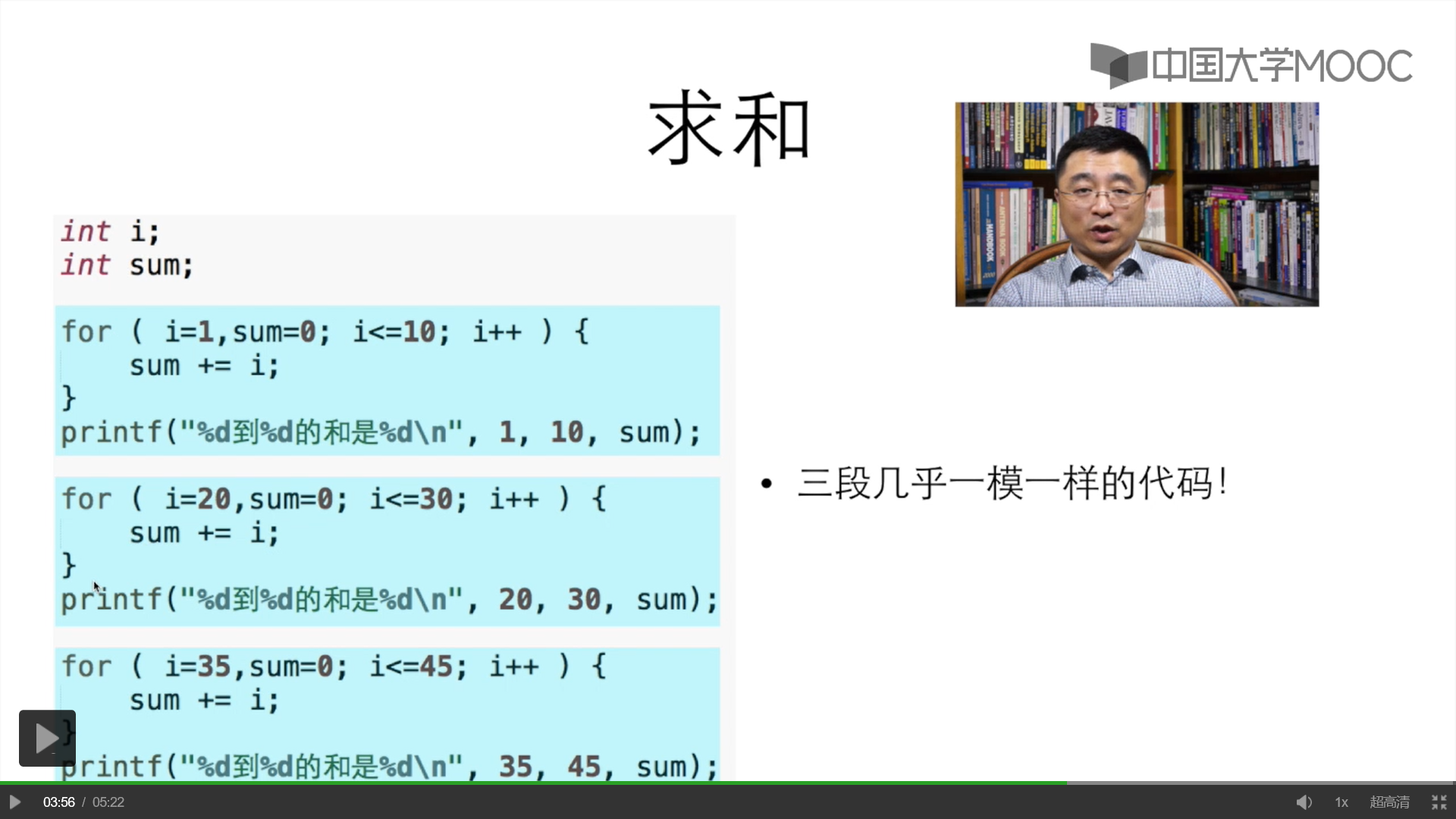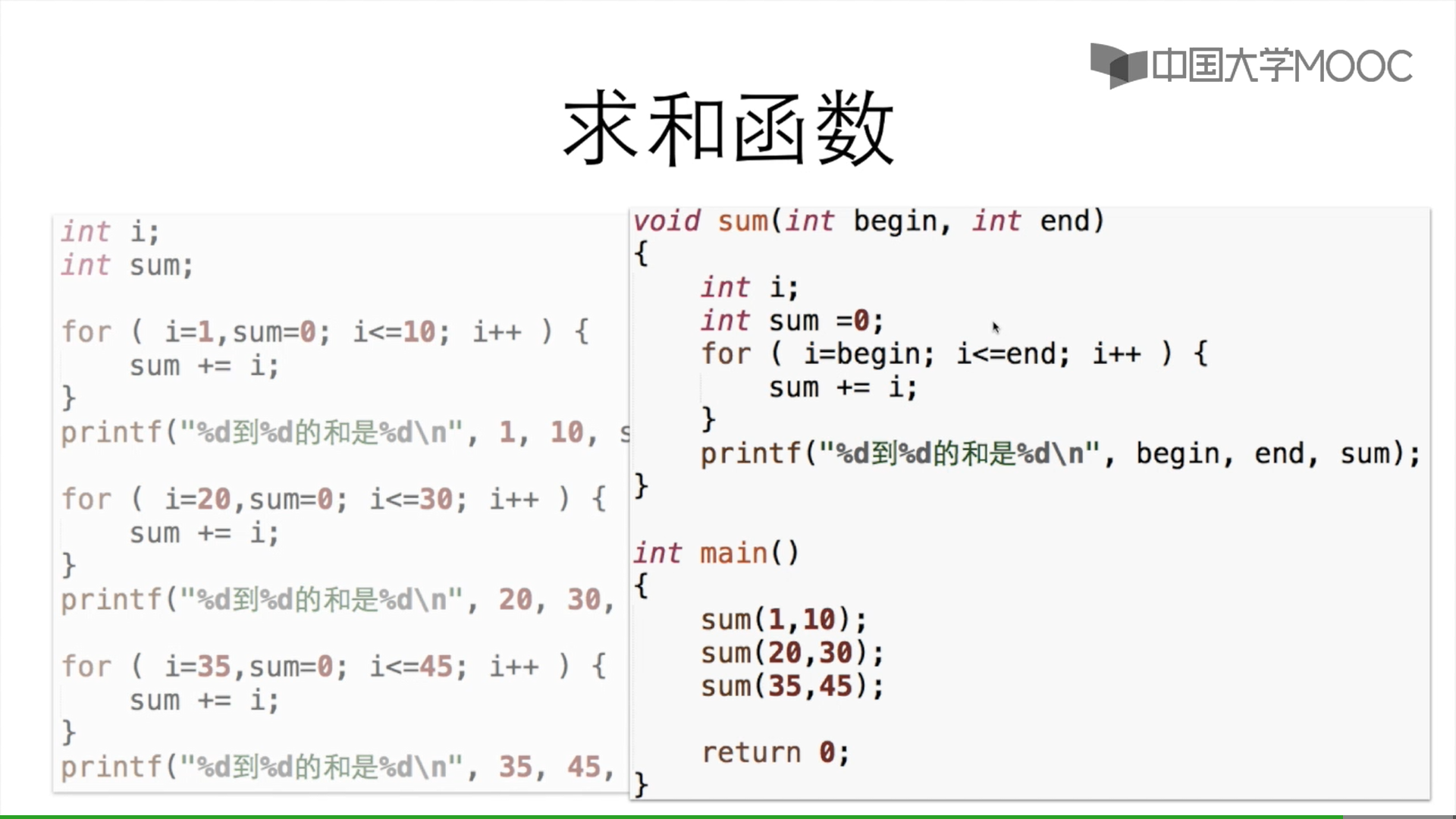通过交换坐标来实现用一个两重循环实现行和列的检查。

3、对于所学知识点暂时没有疑问，但是函数这一章对我来说是难点，本周在复习时要更加注意，同时还应复习pta对应题目帮助自己更好的理解知识点。

【第十六周】

7-2 输出所有大于平均值的数

1、实验代码

#include<stdio.h>
int main(void)
{
int i,n;
double average,sum;
scanf("%d",&n);
int a[n];

sum=0;
if(n>1&&n<=10){
for(i=0;i<n;i++){
scanf("%d",&a[i]);
sum=sum+a[i];
}
average=sum/n;
printf("%.2f\n",average);
for(i=0;i<n;i++){
if(a[i]>average)
printf("%d ",a[i]);
}
}
else{
printf("Invalid.");
}
printf("\n");
return 0;
}

2、解题思路

(1)题目算法：

<=10）来判断是否进入for循环

(2)流程图：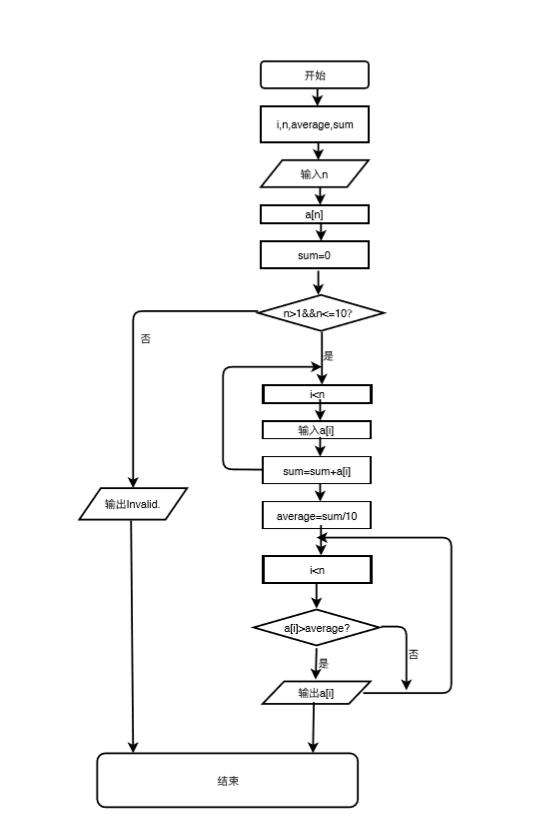3、调试过程遇到的问题及其解决方法：

在输出所有大于平均值的数组时的格式出现问题，当输出的代码为【printf("% d",a[i]);】时，输出的格式就变为【 55 89 78 186】;这边与题目要求的【55 89 78 186】输出格式不符合；因此将输出格式改为【printf("%d ",a[i];)】后输出格式便正确了。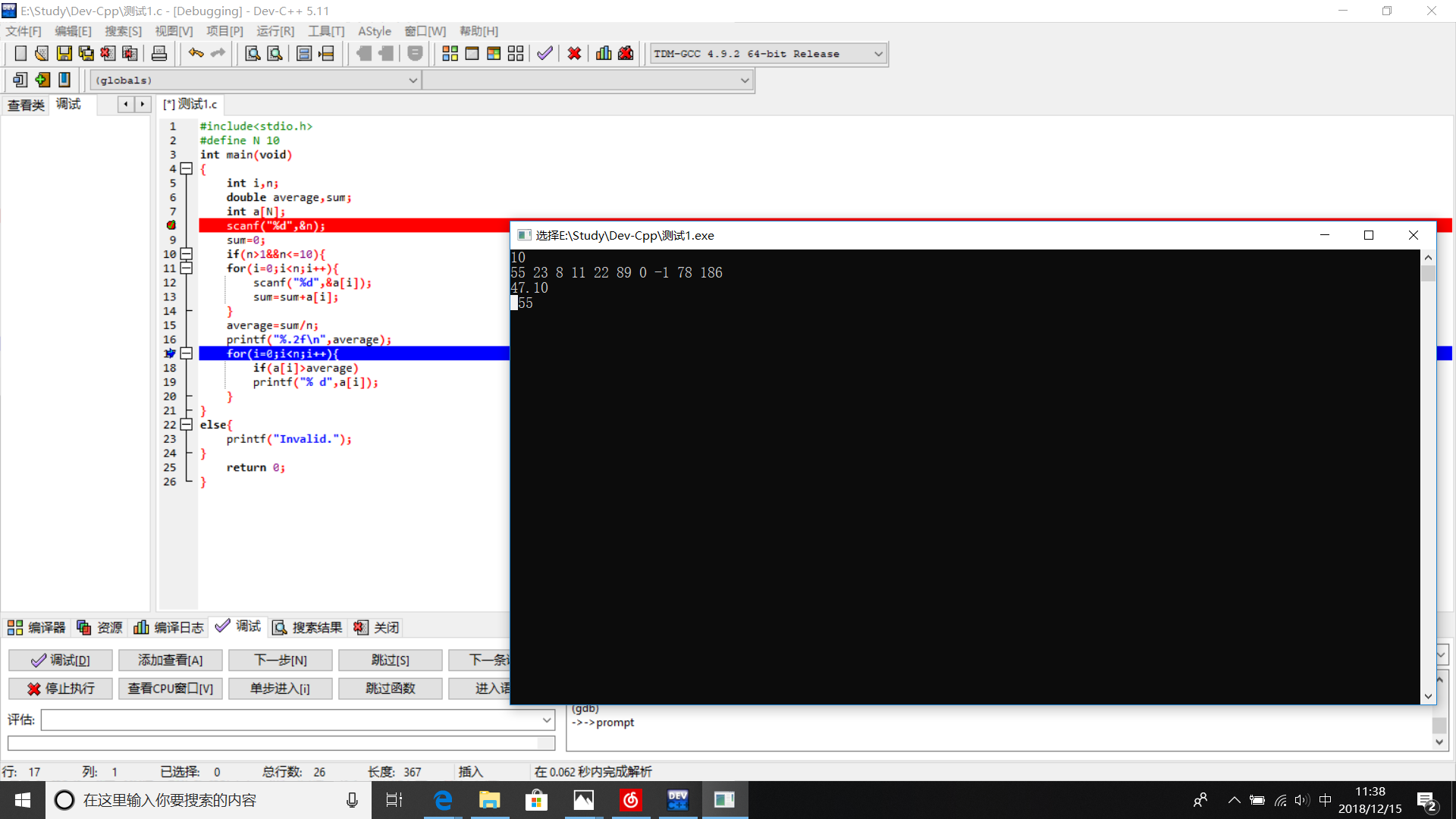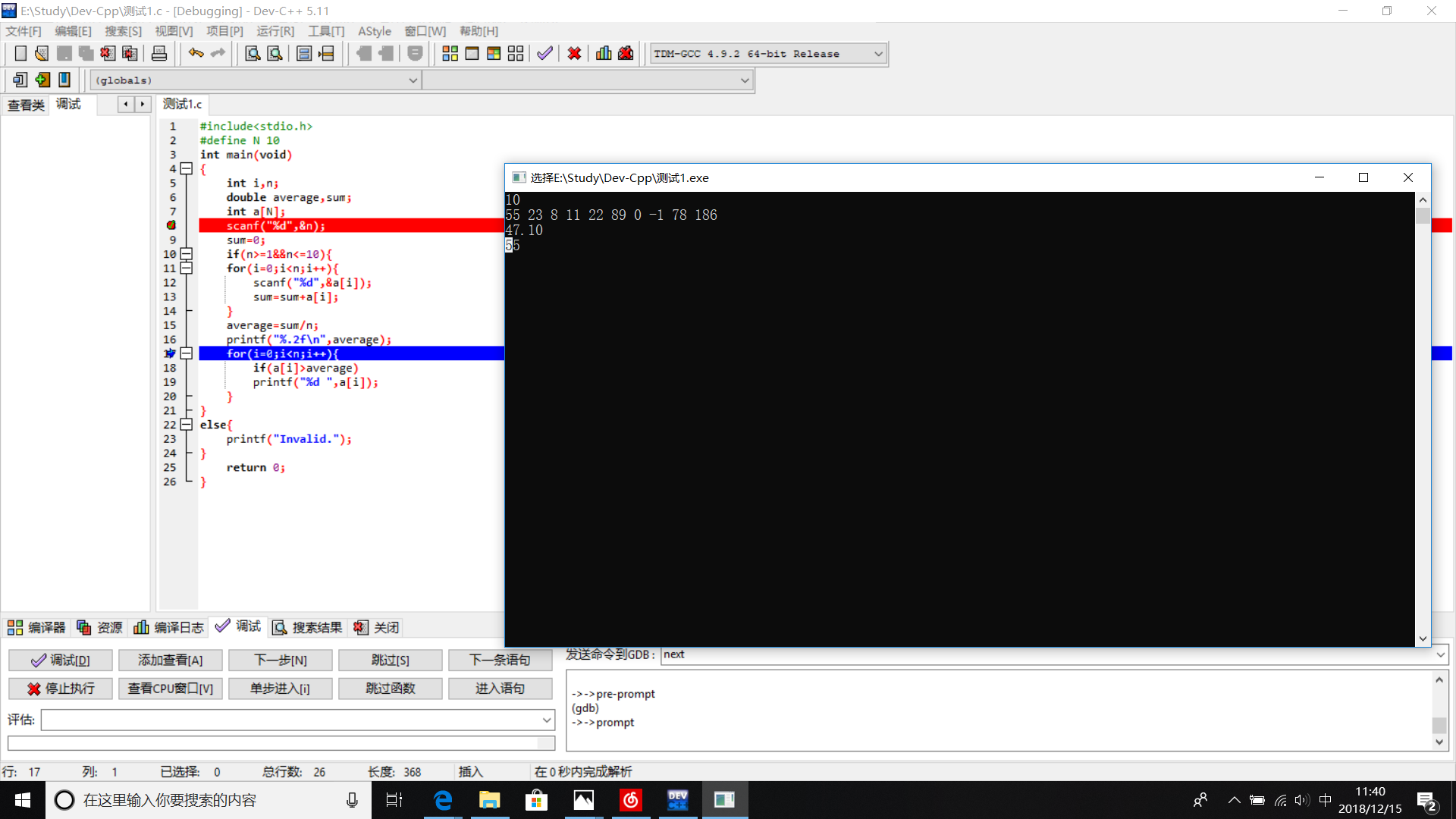另外第二行当输入的n为1时出现的格式错误的情况，在if语句结束后输出【\n】后可得到解决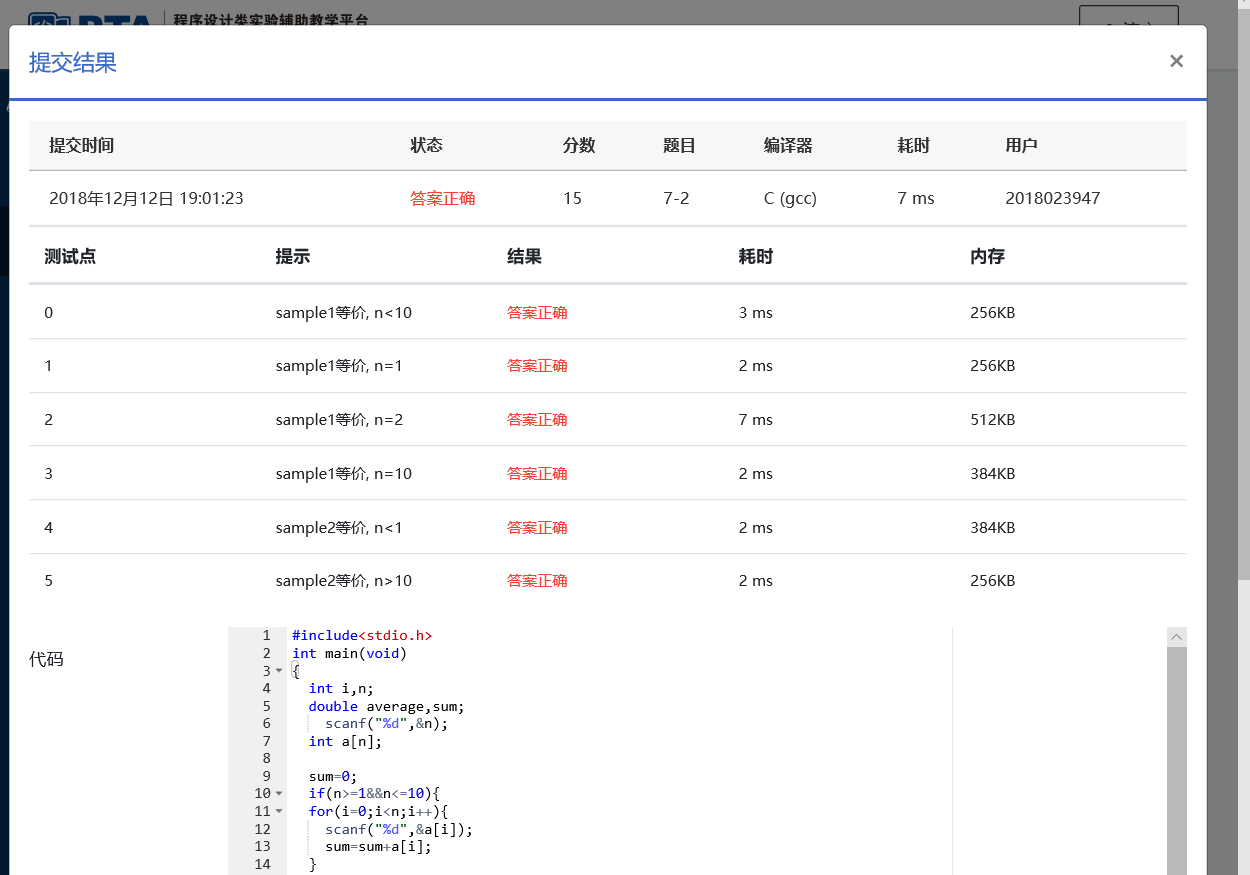—————————————————————————————————————————————

【第十七周】

7-2 求最大值及其下标

1、实验代码

#include<stdio.h>
int main(void){
int i,n,h;
int max;
scanf("%d",&n);
int f[n];
for(i=0;i<n;i++){
scanf("%d",&f[i]);
}
max=f;
h=0;
for(i=0;i<n;i++){
if(max<f[i]){
max=f[i];
h=i;
}
}

printf("%d %d",max,h);
return 0;
}

2、解题思路

(1)题目算法：

给h

(2)流程图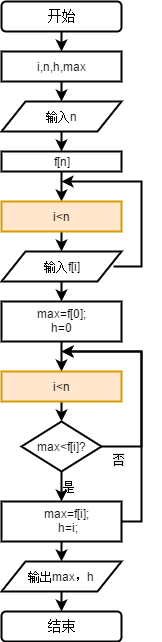3、调试过程中遇到的问题及其解决办法

（附错误程序代码：）

#include<stdio.h>int main(void){  int i,n,h;  int max;  scanf("%d",&n);  int f[n];  for(i=0;i<n;i++){    scanf("%d",&f[i]);  max=f;  h=0;  if(max<f[i]){    max=f[i];    h=i;  }  }  printf("%d %d",max,h);  return 0;}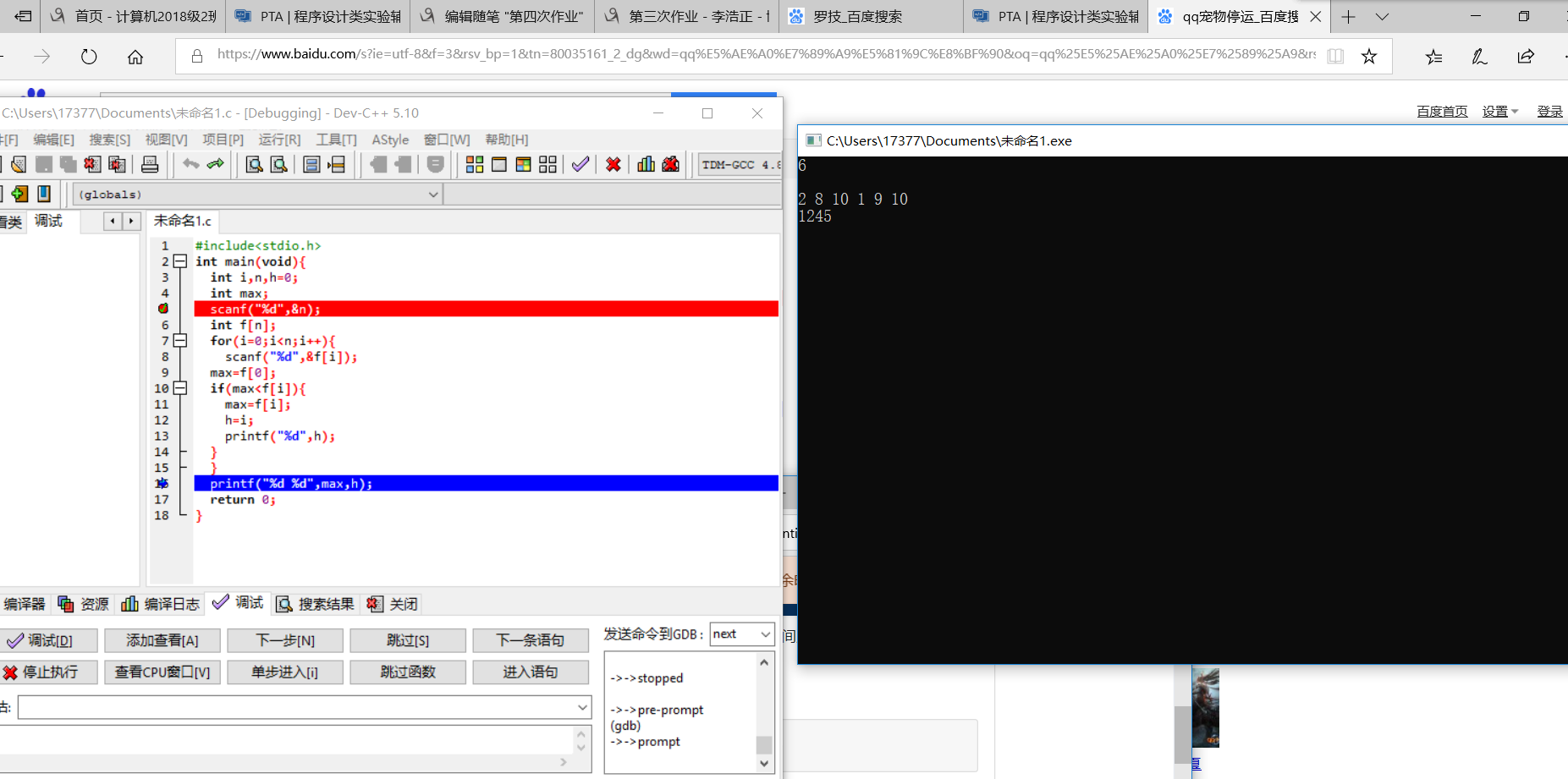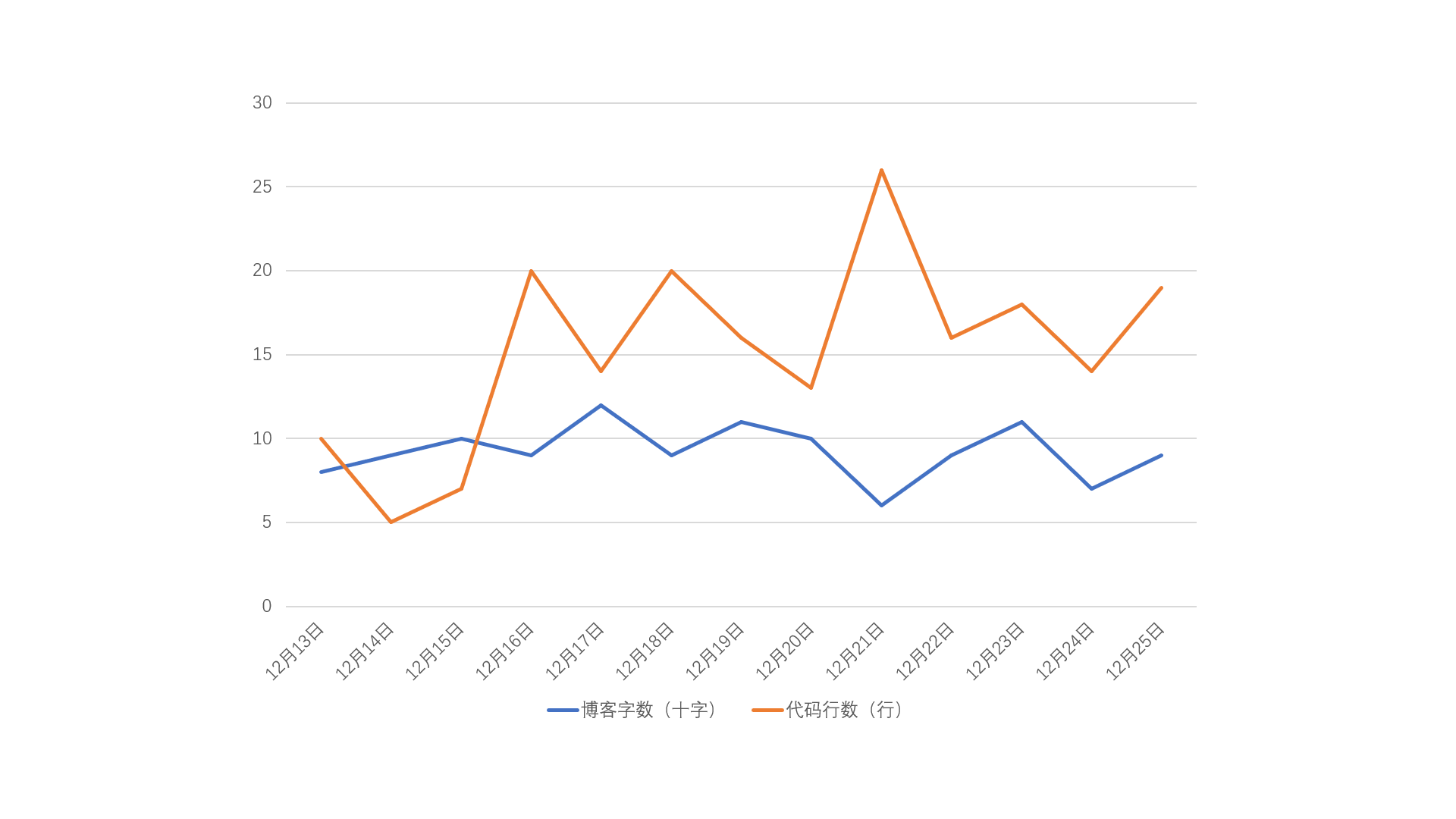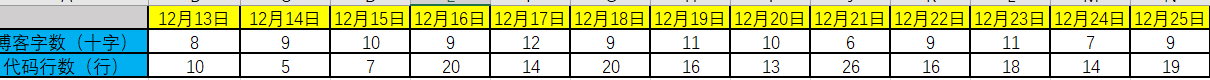个人总结

（3）https://www.cnblogs.com/nansu/p/10134717.html （作者：nansu）

posted @ 2018-12-20 19:08  李浩正  阅读(370)  评论(9编辑  收藏  举报